# Precision measurement of the mass difference between light nuclei and anti-nuclei

The measurement of the mass differences for systems bound by the strong force has reached a very high precision with protons and anti-protons. The extension of such measurement from (anti-)baryons to (anti-)nuclei allows one to probe any difference in the interactions between nucleons and anti-nucleons encoded in the (anti-)nuclei masses. This force is a remnant of the underlying strong interaction among quarks and gluons and can be described by effective theories, but cannot yet be directly derived from quantum chromodynamics. Here we report a measurement of the difference between the ratios of the mass and charge of deuterons and anti-deuterons, and $^{3}{\rm He}$ and $^3\overline{\rm He}$ nuclei carried out with the ALICE (A Large Ion Collider Experiment) detector in Pb-Pb collisions at a centre-of-mass energy per nucleon pair of 2.76 TeV. Our direct measurement of the mass-over-charge differences confirm CPT invariance to an unprecedented precision in the sector of light nuclei. This fundamental symmetry of nature, which exchanges particles with anti-particles, implies that all physics laws are the same under the simultaneous reversal of charge(s) (charge conjugation C), reflection of spatial coordinates (parity transformation P) and time inversion (T).

Figures

## Figure 1

 Examples of squared mass-over-charge ratio distributionsfor deuterons (left) and $\rm{^{3}He}$ (right) in selected rigidity intervals. Particle and anti-particle spectraare in the top and bottom plots, respectively. The fit function (red curve)also includes, for the (anti-)deuteron case, an exponential term to describe the background In the rigidity intervals shown here the background is about 4% for(anti-)deuterons, while it is 0.7% for $^3{\rm He}$ and $^3\overline{\rm He}$.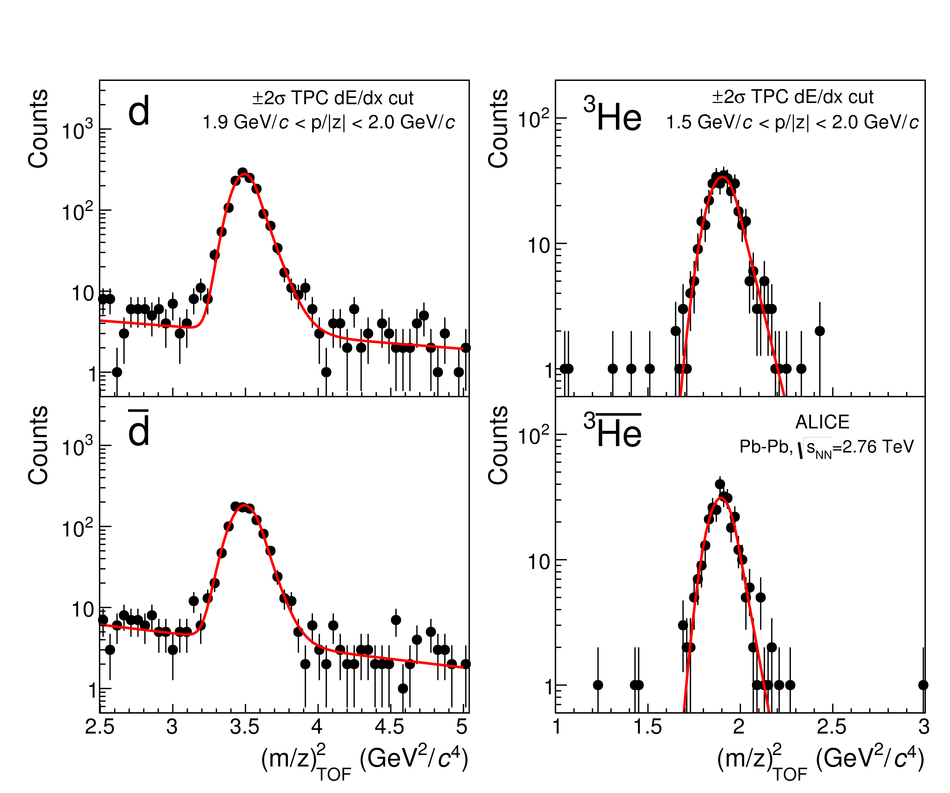## Figure 2

 The $d-\overline{d}$ (top) and $^3{\rm He}-^3\overline{\rm He}$ (bottom) mass-over-charge ratio difference measurements as a function of the particle rigidity. Vertical bars and open boxes show the statistical and the uncorrelated systematic uncertainties (standard deviations), respectively. Both are taken into account to extract the combined result in the full rigidity range, together with the correlated systematic uncertainty, which is shown as a box with tilted lines. Also shown are the $1\sigma$ and $2\sigma$ bands around the central value, where $\sigma$ is the sum in quadrature of the statistical and systematic uncertainties.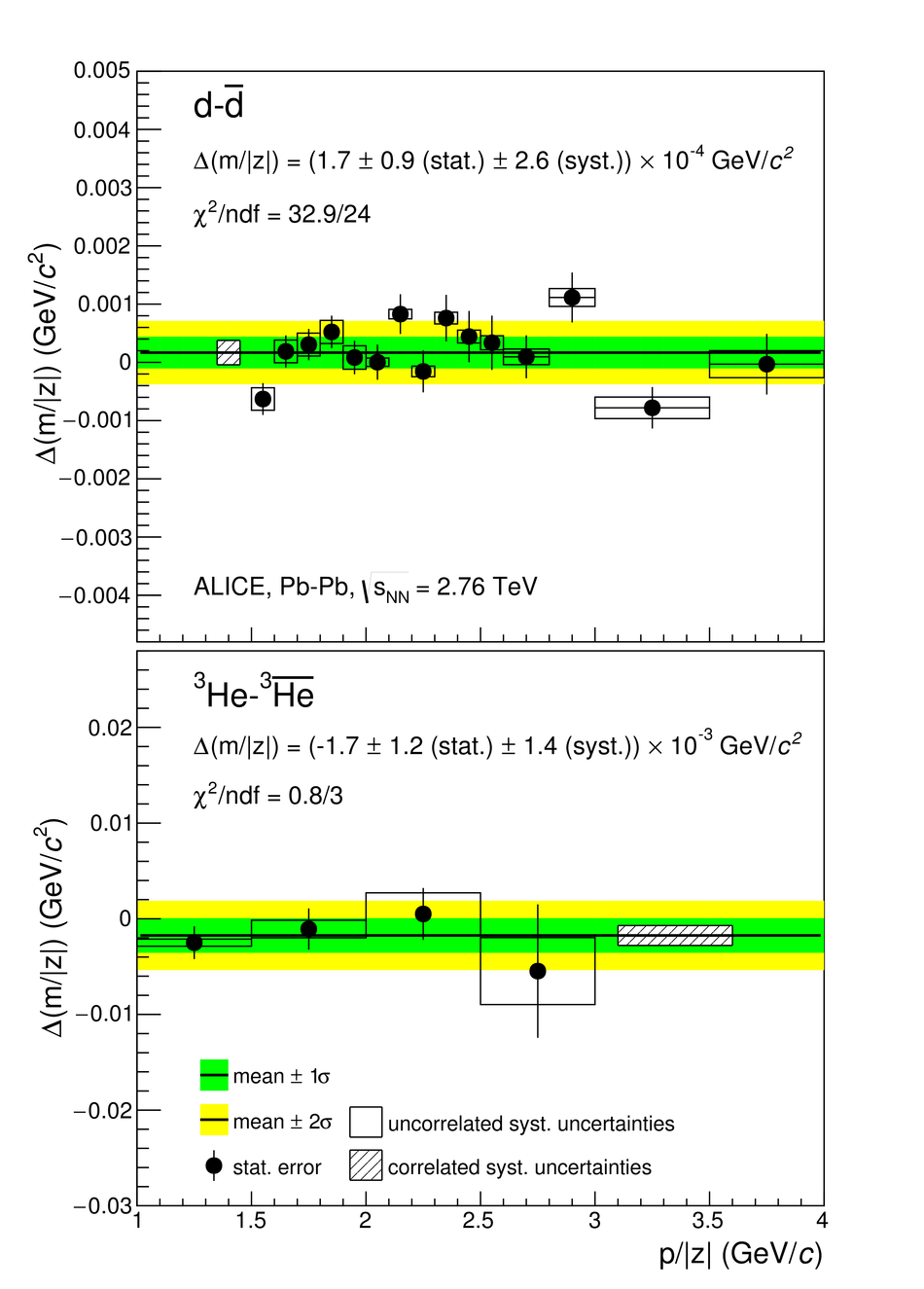## Figure 3

 The ALICE measurements for $d-\overline{d}$ (top) and $^3{\rm He}-^3\overline{\rm He}$ mass-over-charge ratio differences compared with CPT invariance expectation (dotted lines) andexisting mass measurements MAS65, DOR65 and ANT71 (left panel). The inset shows the ALICE results on a finer $\Delta(m/z)/(m/z)$ scale. The right panel shows our determination of the binding energy differences compared with direct measurements from DEN71 and KES99. Error bars represent the sum in quadrature of the statistical and systematic uncertainties (standard deviations).## Figure 1

 Specific energy loss of the tracks ($\rm{d}\mathit{E}/\rm{d}\mathit{x}$) in the TPC versus rigidity for negative and positive particles Lines represent the parameterization of the Bethe-Bloch formula for various particle species(the red lines correspond to (anti-)deuteron and (anti-)$\rm{^{3}He}$ nucleus) Most of the nuclei at low rigidity are produced from the material knock out'':to reject them, the information of the distance of closest approach of the tracks to the reconstructed primary vertex is used (see text)## Figure 2

 Distance of Closest Approach of the tracks to the primary vertexin the transverse plane to the beam direction ($\rm{DCA_{xy}}$) for deuteron (top) and $\rm{^{3}He}$ (bottom) Nuclei and anti-nuclei are shown as empty black points and filled red points, respectively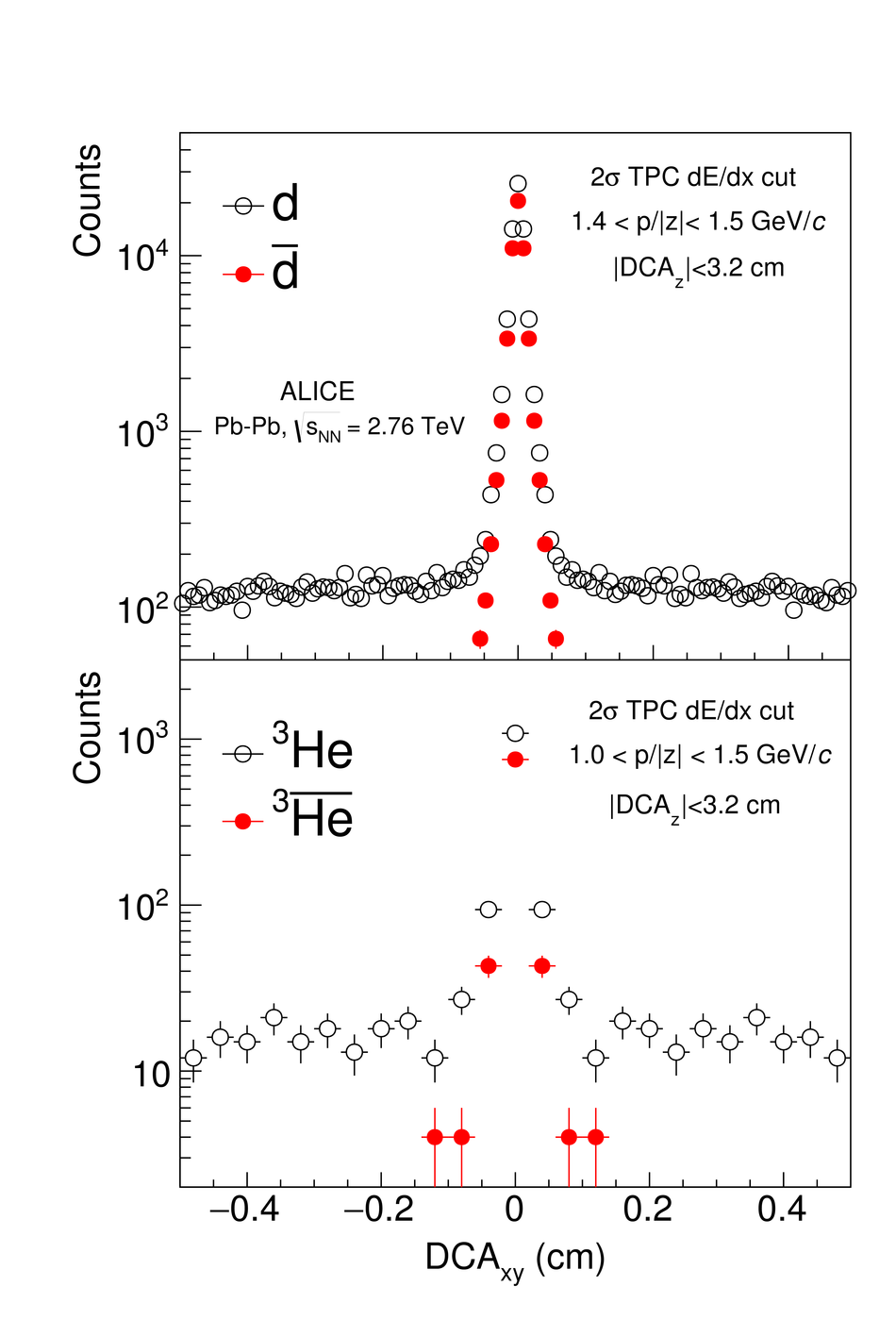## Figure 3

 Ratio between the mean rigidity $\mathcal{h}p/z\mathcal{i}$ and the rigidity at the primary vertex $(p/z)_{\rm{vtx}}$as a function of initial $\beta\gamma=p_{\rm{vtx}}/mc$for deuteron (red filled points) and $^{3}\rm{He}$ (green open points)obtained in the Monte Carlo with the detector simulation. The coloured lines indicate the corresponding parameterizations## Figure 4

 Examples of squared mass-over-charge ratio distributionsfor protons (left), deuterons (centre) and $\rm{^{3}He}$ (right) in selectedrigidity intervals. Particle and anti-particle spectraare in the top and bottom panels, respectively. The fit function (red curve)also includes an exponential term to describe the background In the rigidity intervals shown here the backgroundcorresponds to about 2% for (anti-)protons,and to about 4% for(anti-)deuterons, while it is 0.7% for $\rm{^{3}He}$ and $\rm{^{3}\overline{He}}$.## Figure 5

 Distribution of the mass-over-charge ratio difference measurements of d-$\rm{\overline{d}}$ (top) and $\rm{^{3}He}$-$\rm{^{3}\overline{He}}$ (bottom) obtained in the positive and negative magnetic field configurations (black open points labelled as B(++) and B(--), respectively), by removing the mean rigidity corrections, by varying the fit strategy for (anti-)nuclei and (anti-)protons, the particle selection in the TPC and the requirement on the DCA in the transverse plane and along the beam direction ($\rm{DCA_{xy}}$ and $\rm{DCA_{z}}$, respectively) These are compared to the final result (red and green squares), shown with its statistical uncertainty (vertical bar) and its systematic uncertainty expressed as standard deviation (open box)## Figure 6

 $\rm{d}$-$\rm{\overline{d}}$ (top) and $\rm{^{3}He}$-$\rm{^{3}\overline{He}}$ (bottom) mass-over-charge ratiodifference measurements as a function of the particle rigidity Vertical bars and open boxes show thestatistical and the uncorrelated systematic uncertainties, respectively Both are taken into account to calculate thecombined result in the full rigidity range,together with thecorrelated systematic uncertainty, which is shown as a box with diagonal hatches Also shown are the $1\sigma$ and $2\sigma$ bands around the central value,where $\sigma$ is the sum in quadrature of the statistical and systematic uncertainties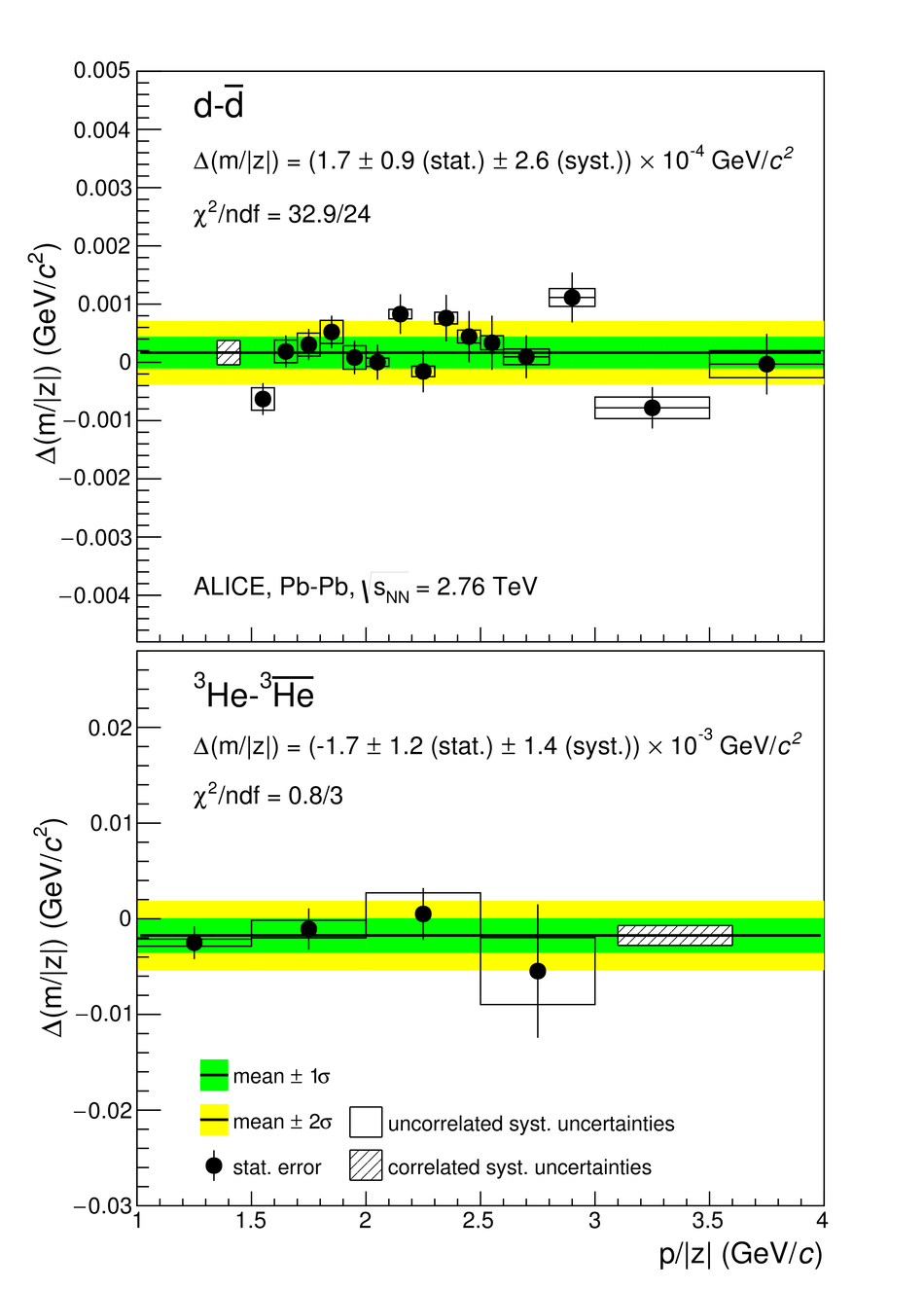## Figure 7

 The ALICE measurements for $\rm{d}$-$\rm{\overline{d}}$ and $\rm{^{3}He}$-$\rm{^{3}\overline{He}}$-over-charge ratio differences compared to CPT invariance expectation (dotted lines) and existing mass measurements (left panel). The inset shows the ALICE results on a finer $\Delta(m/|z|)/(m/|z|)$ scale The right panel shows our determination of the binding energy differencescompared with direct measurements. Error bars represent the sum in quadrature of the statistical and systematic uncertainties.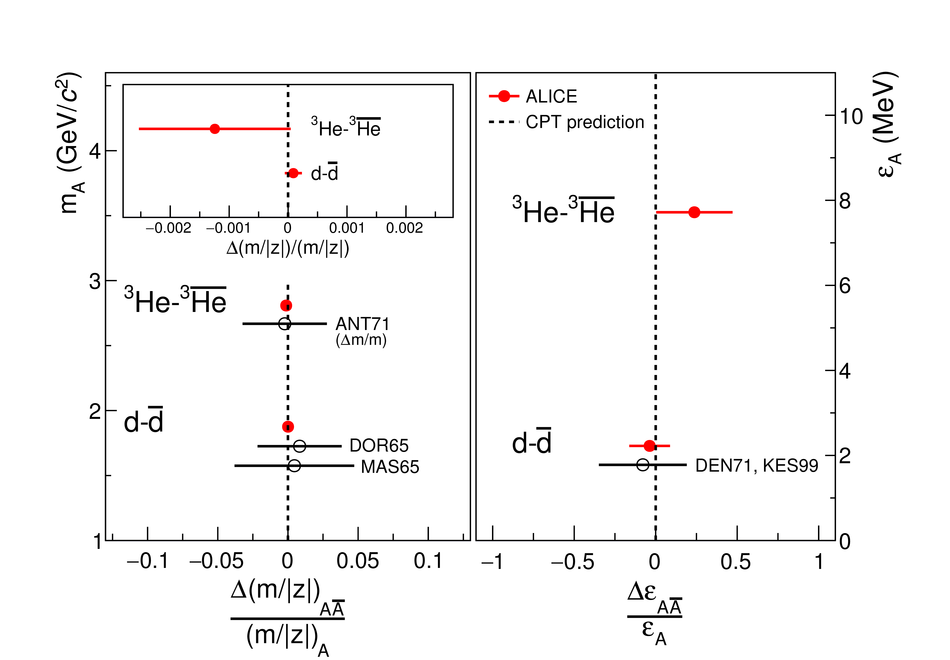## Figure 8

 Experimental limits for CPT invariance $(\rm{CL}=90\%)$ for particles, nuclei and atoms From left to right: measurement of g-factor for the electron and positron ,mass difference between top and anti-top (PDG average),$\rm{W}^{+}-\rm{W}^{-}$, $\rm{\pi}^{+}-\rm{\pi}^{-}$, $\rm{K}^{0}-\rm{\overline{K}}^{0}$ (PDG average), proton and anti-proton, deuteron and anti-deuteron (our result)and the charge of anti-hydrogen